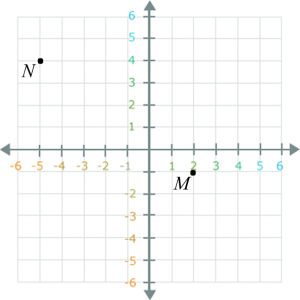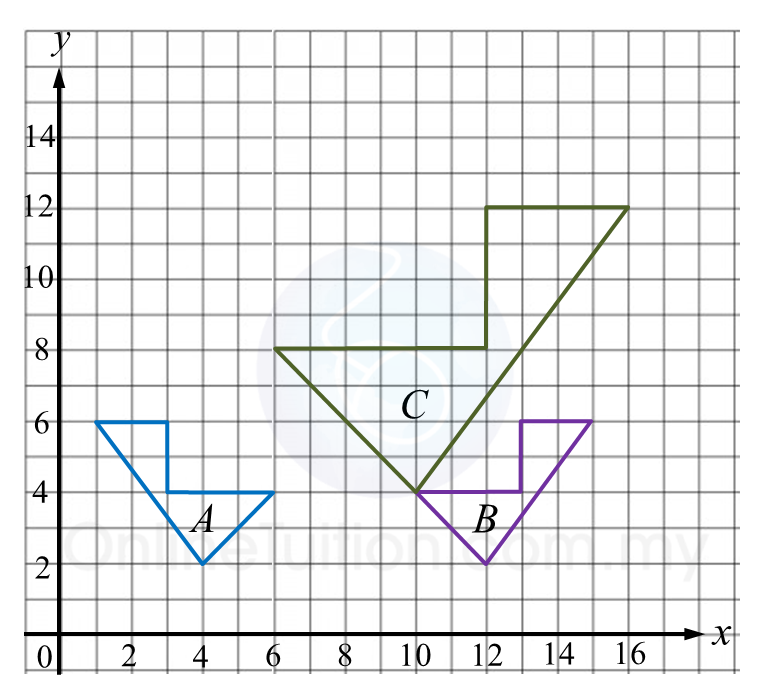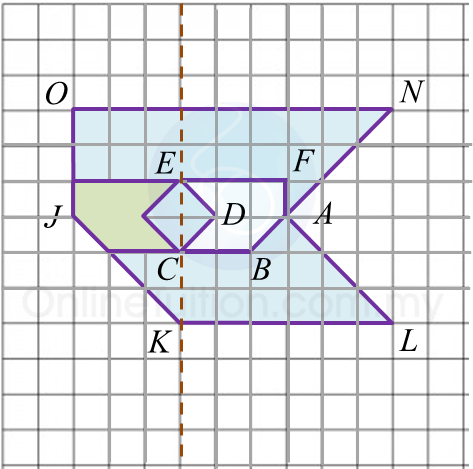# 3.3.3 Transformation III, SPM Paper 2 (Long Questions)

Question 5:
(a) Diagram below shows two points, M and N, on a Cartesian plane.Transformation T is a translation and transformation R is an anticlockwise rotation of 90o about the centre (0, 2).
(i) State the coordinates of the image of point M under transformation R.
(ii) State the coordinates of the image of point N under the following transformations:
(a) T2,
(b) TR,

(b) Diagram below shows three pentagons, A, B and C, drawn on a Cartesian plane.(i) C is the image of A under the combined transformation WV.
Describe in full the transformation:
(a) V    (b) W
(ii) Given A represents a region of area 12 m2, calculate the area, in m2, of the region represented by C.

Solution:
(a)(b)(b)(i)(a)
V: A reflection in the line x  = 8

(b)(i)(b)
W: An enlargement of scale factor 2 with centre (14, 0).

(b)(ii)
Area of B = area of A = 12 m2
Area of C = (Scale factor)2 x Area of object
= 22 x area of B
= 22 x 12
= 48 m2

Question 6:
(a) Diagram below shows point M marked on a Cartesian plane.Transformation T is a translation $\left(\begin{array}{l}\text{2}\\ 3\end{array}\right)$ and transformation R is an anticlockwise rotation of 90o about the centre O.
State the coordinates of the image of point M under each of the following transformations:
(i) RT,
(ii) TR,

(b) Diagram below shows two hexagons, ABCDEF and JKLANO, drawn on square grids.(i) JKLANO is the image of ABCDEF under the combined transformation VW.
Describe in full the transformation:
(a) W   (b) V
(ii) It is given that quadrilateral ABCDEF represents a region of area 45 m2.
Calculate the area, in m2, of the region represented by the shaded region.

Solution:
(a)(b)(i)(a)
V: A reflection in the line EC.

(i)(b)

(ii)
Area of JKLANO
= 32 x area of ABCDEF
= 9 x 45
= 405 m2

Area of the coloured region
= area of JKLANO – area of ABCDEF
= 405 – 45
= 360 m2